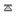## Random number function

From html only
This function will produce a random number between val1 and val2 inclusive

<xt:random from="val1" to="val2" />

Multiple calls on one page
using the same input values
will produce the same output.
<xt:random from="0" to="10000"/>
1st call 4850
2nd call 4850

To cure this add another operator/argument pair I'm using x
<xt:random from="0" to="10000" x="1"/>
3838
Change the value of x to change the output.
<xt:random from="0" to="10000" x="2"/>
6656

The value of x can be almost anything
<xt:random from="0" to="10000" x="hello"/>
8562
###### Also see
Countdown Device type Gen time file count Refefer url Current urlAbout us Contact Forum